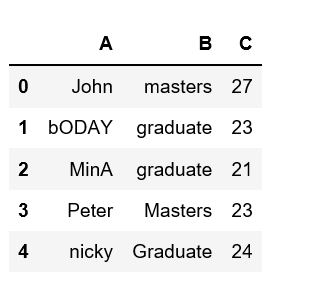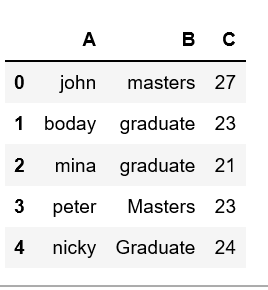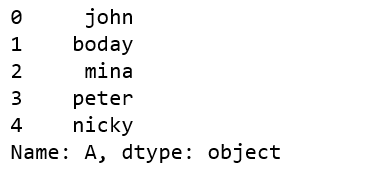# How to lowercase strings in a column in Pandas dataframe

Analyzing real-world data is somewhat difficult because we need to take various things into consideration. Apart from getting the useful data from large datasets, keeping data in required format is also very important. One might encounter a situation where we need to lowercase each letter in any specific column in given dataframe. Let’s see how to lowercase column names in Pandas dataframe. Let’s create a dataframe from the dict of lists.

## Python3

 `# Create a simple dataframe` ` `  `# importing pandas as pd` `import` `pandas as pd`   `# creating a dataframe` `df ``=` `pd.DataFrame({``'A'``: [``'John'``, ``'bODAY'``, ``'MinA'``, ``'Peter'``, ``'nicky'``],` `                  ``'B'``: [``'masters'``, ``'graduate'``, ``'graduate'``,` `                                   ``'Masters'``, ``'Graduate'``],` `                  ``'C'``: [``27``, ``23``, ``21``, ``23``, ``24``]})` ` `  `df`

Output:There are certain methods we can change/modify the case of column in pandas dataframe. Let’s see how can we lowercase column names in Pandas dataframe using lower() method. Method #1:

## Python3

 `# Create a simple dataframe` ` `  `# importing pandas as pd` `import` `pandas as pd`   ` `  `# creating a dataframe` `df ``=` `pd.DataFrame({``'A'``: [``'John'``, ``'bODAY'``, ``'MinA'``, ``'Peter'``, ``'nicky'``],` `                  ``'B'``: [``'masters'``, ``'graduate'``, ``'graduate'``, ` `                                   ``'Masters'``, ``'Graduate'``],` `                  ``'C'``: [``27``, ``23``, ``21``, ``23``, ``24``]})` ` `  `df[``'A'``] ``=` `df[``'A'``].``str``.lower()`   `df`

Output:Method #2:

## Python3

 `# Create a simple dataframe` ` `  `# importing pandas as pd` `import` `pandas as pd`   ` `  `# creating a dataframe` `df ``=` `pd.DataFrame({``'A'``: [``'John'``, ``'bODAY'``, ``'MinA'``, ``'Peter'``, ``'nicky'``],` `                  ``'B'``: [``'masters'``, ``'graduate'``, ``'graduate'``,` `                                   ``'Masters'``, ``'Graduate'``],` `                  ``'C'``: [``27``, ``23``, ``21``, ``23``, ``24``]})` ` `  `df[``'A'``].``apply``(``lambda` `x: x.lower())`

Output:Whether you're preparing for your first job interview or aiming to upskill in this ever-evolving tech landscape, GeeksforGeeks Courses are your key to success. We provide top-quality content at affordable prices, all geared towards accelerating your growth in a time-bound manner. Join the millions we've already empowered, and we're here to do the same for you. Don't miss out - check it out now!

Previous
Next